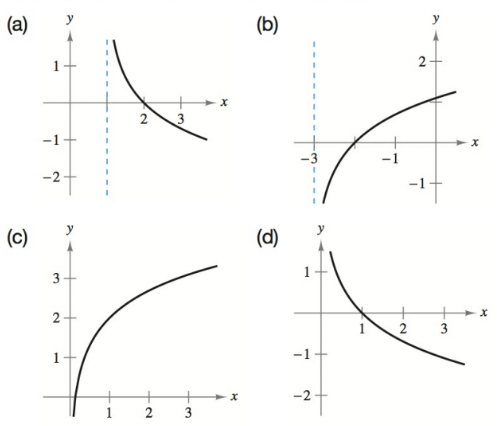Chapter 4.4, Problem 12E### Calculus: An Applied Approach (Min...

10th Edition
Ron Larson
ISBN: 9781305860919

#### Solutions

Chapter
Section### Calculus: An Applied Approach (Min...

10th Edition
Ron Larson
ISBN: 9781305860919
Textbook Problem
1 views

# Matching In Exercises 9-12, match the function with its graph. [The graphs are labeled (a)—(d).]f ( x ) = − ln ( x − 1 )

To determine

The correct match of the function f(x)=ln(x1) from the provided graphs,

Explanation

Given Information:

The provided function is f(x)=ln(x1) and the provided graphs are,

Consider the function, f(x)=ln(x1)

The natural logarithmic function is defined only for positive values, so the domain of the function, f(x)=ln(x1) is x1>0 or x>1. Then, the vertical asymptote of the function is x=1 as the function f(x) only gives value for x>1.

Compute the value of f(x) at x=2 as,

f(2)=ln(2

### Still sussing out bartleby?

Check out a sample textbook solution.

See a sample solution

#### The Solution to Your Study Problems

Bartleby provides explanations to thousands of textbook problems written by our experts, many with advanced degrees!

Get Started

#### Find more solutions based on key concepts